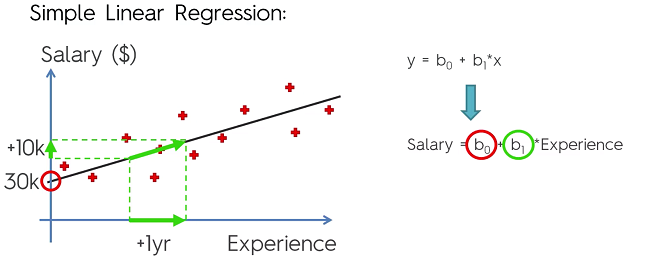## Introduction to Simple Linear Regression

Formula: $y= b_{_0} + b_{_1}x_{_1}$

Basically, the formula for a straight line with:

• $y$ : Dependent Variable (DV)
• $b_{_0}$ : Constant
• $b_{_1}$ : Coefficient of IV. Represents how a unit change in $x_{_1}$ effects a unit change in $y$.
• $x_{_1}$ : Independent Variable (IV)

Simple Linear Regression is a method of finding a line that best fits a set of data points on the graph. The methodology in getting the line of best fit is simple. Let’s say we drew a vertical line from all our points to our guess line. The distance can be written $y_{_i}$ where i represents the point number. For the line to be the line of best fit is if the value of the following formula is the lowest it can possibly be:

$sum$ ($y_{_i}$ - $y_{_r})^2$ –> min

Called the Orderly Least Square Method## Creating a simple Linear Regression in Python

First we need to preprocess the data using the preprocessing template:

#Importing the libraries
import numpy as np
import matplotlib.pyplot as plt
import pandas as pd

#Importing the dataset
X = dataset.iloc[:, :-1].values
y = dataset.iloc[:, 3].values

#Taking care of missing data
from sklearn.preprocessing import Imputer
imputer = Imputer(missing_values = 'NaN', strategy = 'mean', axis = 0)
imputer = imputer.fit(X[:, 1:3])
X[:, 1:3] = imputer.transform(X[:, 1:3])

#Encoding categorical data
#Encoding the Independent Variable
from sklearn.preprocessing import LabelEncoder, OneHotEncoder
labelencoder_X = LabelEncoder()
X[:, 0] = labelencoder_X.fit_transform(X[:, 0])
onehotencoder = OneHotEncoder(categorical_features = )
X = onehotencoder.fit_transform(X).toarray()
#Encoding the Dependent Variable
labelencoder_y = LabelEncoder()
y = labelencoder_y.fit_transform(y)

#Splitting the dataset into the Training set and Test set
from sklearn.cross_validation import train_test_split
X_train, X_test, y_train, y_test = train_test_split(X, y, test_size = 0.2, random_state = 0)

#Feature Scaling
from sklearn.preprocessing import StandardScaler
sc_X = StandardScaler()
X_train = sc_X.fit_transform(X_train)
X_test = sc_X.transform(X_test)
sc_y = StandardScaler()
y_train = sc_y.fit_transform(y_train)


Note: We do not to perform feature scaling, the scikit learn library will handle that for us.

Import the LinearRegression library from SciKit-Learn.

from sklearn.linear_model import LinearRegression


Create object of class and fit it to the training set

regressor = LinearRegression()
regressor = regressor.fit(X_train, y_train)


Now we need to create a vector that contains the predictions of the test set. (Contains predicted values of test set. With the matrix of text set X.

y_pred = regressor.predict(X_test)


So y_pred will be out predicted results and y_test will be our actual results.